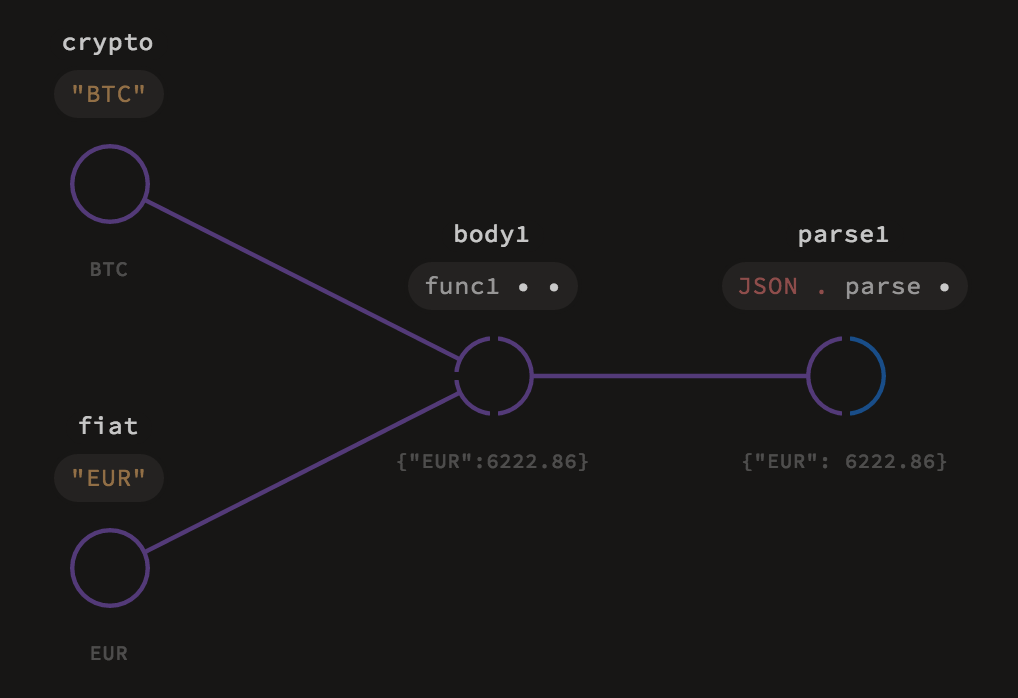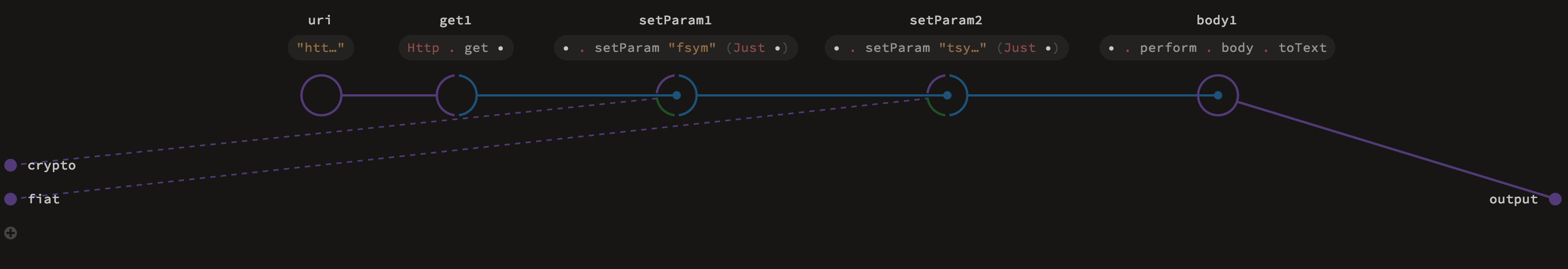# Lambda

``````id = x: x
const = x: y: x
myLambda = x: y: z: (x + y) * z
``````

``````_.succ # 同(x: x.succ)
_.toText + _.toText # 同(x: y: x.toText + y.toText)
``````

# 模块视图# 函数定义